# MP Board Class 11th Chemistry Solutions Chapter 10 The s-Block Elements

In this article, we will share MP Board Class 11th Chemistry Solutions Chapter 10 The s-Block Elements Pdf, These solutions are solved by subject experts from the latest edition books.

## MP Board Class 11th Chemistry Solutions Chapter 10 The s-Block Elements

### MP Board Class 11 Chemistry The s-Block Elements Textbook Questions and Answers

Question 1.
What are the common physical and chemical features of alkali metals?
Solution.
Refer to section 10.1.

Question 2.
Discuss the general characteristics and gradation in properties of alkaline earth metals.
Solution.
Refer to section 10.6.

Question 3.
Solution.
Alkali metals are highly reactive and hence they do not occur in the free state.

Question 4.
Find the oxidation state of sodium in Na2O2.
Solution.
Let x be the oxidation state of Na in Na202. Since Na202 contains a peroxide . linkage in which O has an oxidation state of – 1, therefore,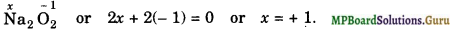Question 5.
Explain why sodium is less reactive than potassium?
Solution.
The ionization enthalpy of potassium is less than that of sodium and hence potassium is more reactive than sodium.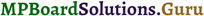Question 6.
Compare the alkali metals and alkaline earth metals with respect to
(i) ionization enthalpy (ii) basicity of oxides and (iii) solubility of hydroxides.
Solution.
(i) Ionization enthalpy. Because of higher nuclear charge and smaller atomic size, the ionization enthalpies of alkaline earth metals are higher than those of the corresponding alkali metals.

(ii) Basicity of oxides. Since the ionization enthalpy of alkali metals is less or the electropositive character of alkali metals is more than that of the corresponding alkaline earth metals, therefore alkali metal oxides are more basic than the corresponding alkaline earth metal oxides.

(iii) Solubility of hydroxides. Due to smaller size and higher ionic charge, the lattice enthalpies of alkaline earth metals hydroxides are much higher than those of alkali metals and hence the solubility of alkali metal hydroxides is more than that of alkaline earth metal hydroxides.

Question 7.
In what ways does lithium show similarities to magnesium in its chemical behaviour?
Solution.
Points of similarities between lithium and magnesium:
(i) Li and Mg are harder and lighter.
(ii) Li and Mg react slowly with cold water.
(iii) Li2O and MgO are much less soluble and their hydroxides decompose at red hot temperature.(iv) Li and Mg react with N2 to form nitrides
6Li + N2 →2Li3N
3Mg + N2 → Mg3N2.
(a) Both cannot form peroxides and superoxides.
(vi) Carbonates of both are covalent and decompose on heating.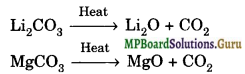(vii) Solid Dicarbonates are not formed by Li and Mg.
(viii) Both LiCl and MgCl2 are soluble in ethanol.
(ix) Both LiCl and MgCl2 are deliquescent.
(x) Behave as a weak base.

Question 8.
Explain why alkali and alkaline earth metals cannot be obtained by chemical reduction methods?
Solution.
Alkali and alkaline earth metals are themselves very strong reducing agents and reducing agents stronger than them are not easily available. Therefore, these metals cannot be obtained by chemical reduction methods.

Question 9.
Why are potassium and caesium, rather than lithium used in photoelectric cells?
Solution.
Potassium and caesium have much lower ionization enthalpy than that of lithium. As a result, these metals on exposure to light, lose electrons much more easily than lithium. Hence, potassium and caesium rather than lithium are used in photoelectric cells.

Question 10.
When an alkali metal dissolves in liquid ammonia, the solution acquires different colours. Explain the reasons for this type of colour change.
Solution.
When an alkali metal is dissolved in liquid ammonia, it produces a blue coloured conducting solution due to formation of ammoniated cation and ammoniated electron as given below: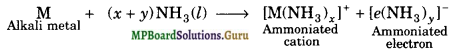When the concentration is above 3M, the colour of solution is copper-bronze. This colour change is because the concentrated solution contains clusters of metal ions and hence possesses metallic lustre.

Question 11.
Beryllium and magnesium do not give colour to flame whereas other alkaline earth metals do so. Why ?
Solution.
Due to the small size, the ionization enthalpies of Be and Mg are much higher than those of other alkaline earth metals. This means that the valence electrons in beryllium and magnesium are more tightly held by the nucleus. Therefore, they need large amount of energy for excitation of electrons to higher energy levels. Since such a large amount of energy is not available in bunsen flame, therefore, these metals do not impart any colour to the flame.

Question 12.
Discuss the various reactions that occur in the Solvay ammonia process.
Solution.
In Solvay ammonia process, in the carbonation tower CO2 is passed through brine, saturated with ammonia when sodium bicarbonate is formed, which being sparingly soluble gets precipitated.
NaCl + NH3 + CO2 + H2O → NaHCO3↓ + NH4Cl ………….. (i)
Sodium bicarbonate is separated which on heating gives sodium carbonate.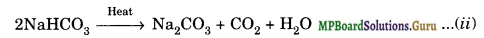CO2 needed for the reaction is prepared by heating limestone and the quick lime, CaO is treated with water to form slaked lime, Ca(OH)2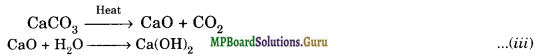NH3 is recovered from filtrate of reaction (i) by heating with Ca(OH)2
2NH4Cl + Ca(OH)2 → 2NH3 + CaCl2 + 2H2O

Question 13.
Potassium carbonate cannot be prepared by Solvay process. Why?
Solution.
Potassium carbonate cannot be prepared by Solvay process because potassium bicarbonate being highly soluble in water does not get precipitated in carbonation tower when CO2 is passed through a concentrated solution of KCl saturated with ammonia.

Question 14.
Why Li2CO3 decomposes at lower temperature whereas Na2CO3 at higher temperature?
Solution.
Li+ ion is smaller in size. It forms a more stable lattice with smaller anion oxide, O2 than with CO32- ion. Therefore, Li2CO3 decomposes into Li2O at lower temperature. On the other hand, Na+ ion being larger in size forms a more stable lattice with larger anion CO32- than with O2- ion. Therefore, Na2CO3 is quite stable and decomposes into Na2O at very high temperature.Question 15.
Compare the solubility and thermal stability of the following compounds of the alkali metals with those of alkaline earth metals (a) nitrates (b) carbonates (c) sulphates.
Solution.
Thermal Stabilities
Group-1 metal compounds are relatively more stable to heat whereas the salts of group-2 are less stable.
(a) Nitrates: Nitrates of alkali metals, except LiNO3, decompose on strong heating forming nitrites and oxygen. For example,
2KNO3(S) → 2KNO2(S) + O2(g)
Nitrates of alkaline-earth metals and LiN03 decompose on heating to form oxides, nitrogen dioxide and oxygen.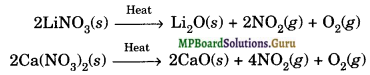Thermal stabilities of nitrates of group-1 and group-2 metals increase on moving down the group from top to bottom.

(b) Carbonates and Hydrogencarbonates: The carbonates of alkali metals except lithium carbonate are stable to heat. The carbonates of group-2 metals and that of lithium decompose on heating, forming an oxide and carbon dioxide. For example,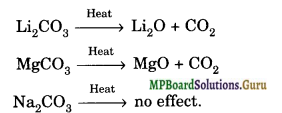The stabilities of carbonates of alkaline earth metals increase on moving down the group. For example, BeCO3 decomposes at 373 K, MgCO3 at 813 K, CaCO3 at 1173 K, SrCO3 at 1563 K and BeCO3 at 1633 K.
Lithium and group-2 metals do not form solid hydrogen carbonates, although they exist in solution. On heating these solutions, the hydrogen carbonates decompose to form carbonates and CO2 gas is liberated. The solid. hydrogen carbonates of alkali metals decompose between 375 and 575 K.
2NaHCO3(s) → Na2CO3(s) + H2O(g) + CO2(g)
(c) Sulphates: The sulphates of group-1 and group-2 metals are all thermally stable.

Solubilities in Water
(a) Nitrates: Nitrates of group-1 and group-2 metals are all soluble in water. The solubilities of these salts further increase on descending the group.

(b) Carbonates and Hydrogencarbonates: Carbonates of alkali metals, except Li2CO3, are soluble in water. Their solubilities increase on moving down the group. Carbonates of group-2 metals are almost insoluble in water and their solubilities further decrease on moving down the group. In fact, these metals can be precipitated from their salt solutions as carbonates. Hydrogen carbonates of group-1 and group-2 metals are soluble in water.

(c) Sulphates: Sulphates of alkali metals are soluble in water. The sulphates of alkaline earth metals are less soluble. Their solubilities decrease on moving down the group from Be to Ba.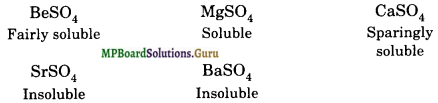Question 16.
Starting with sodium chloride how would you prepare (i) sodium metal (ii) sodium hydroxide (iii) sodium peroxide and (iv) sodium carbonate.
Solution.
(i) Sodium metal: By electrolysis of sodium chloride. During the electrolysis, the molten metal liberated at the cathode moves up and flows into the receiver containing Kerosene oil. The following reactions take place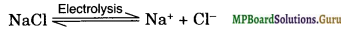at cathode: Na+ +e → Na
at anode: Cl → Cl + e
Cl + Cl → Cl2

(ii) Sodium hydroxide is manufactured by the electrolysis of NaCl solution (brine) in a specially designed cell called Castner Kellner cell.
NaCl →Na+ + Cl
At cathode: Na+ +e → Na
Sodium dissolves in mercury to form sodium amalgam which due to raking motion of the cell travels to the central compartment.
At anode: Cl → Cl + e
Cl + Cl → Cl2
Central compartment: In this compartment sodium amalgam acts as anode by induction.
NaOH → Na+ + OH
At cathode: Na+ + e → Na
Na + H2O → NaOH +  H2
So, at cathode hydrogen gas is liberated and sodium hydroxide is formed.

(iii) NaCl + NH4HCO3 → NaHCO3+ NH4
Sodium hydrogen carbonate so formed precipitates out because of the common ion effect caused due to the presence of excess of sodium chloride. The precipitated NaHCO3 is filtered off and then ignited to get sodium carbonate.
2NaHCO3 → Na2CO3 + CO2 + H2O

Question 17.
What happens when
(i) Magnesium is burnt in air
(ii) Quick lime is heated with silica
(iii) Chlorine reacts with slaked lime
(iv) Calcium nitrate is heated?
Solution.
(i) Magnesium reacts with air to form a mixture of MgO and magnesium nitride.
2Mg + O2→ 2MgO
3Mg + N2→ (Magnesium nitride)
(ii) On heating quick lime with silica, calcium silicate is formed.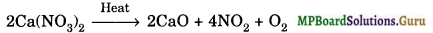(iii) The reaction of chlorine with slaked lime forms bleaching powder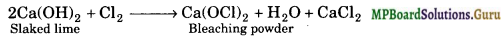(iv) On heating, calcium nitrate decomposes to give NO2 gas along with O2 gas.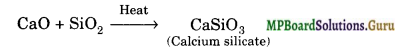Question 18.
Describe two important uses of the following:
(i) Caustic soda,
(ii) Sodium carbonate
(iii) Quick lime.
Solution.

(i) Caustic soda:

• It is used in the manufacture of paper, soap and artificial silk.
• It is used for the preparation of sodium metal and many salts of sodium.

(ii) Sodium carbonate:

• It is used for softening hard water.
• It is used in paper, paints and textile industries.

(iii) Quick lime:

• It is used in the purification of sugar.
• It is used in drying of gases and alcohol.

Question 19.
Draw the structure of (i) BeCl2 (vapour) (ii) BeCl2 (solid).
Solution.
In the vapour state, it exists as a dimer.In the solid state, BeCl2 has polymeric structure with chlorine bridges.Question 20.
The hydroxides and carbonates of sodium and potassium are easily soluble in water while the corresponding salts of magnesium and calcium are sparingly soluble in water. Explain.
Solution.
Thermal Stabilities
Group-1 metal compounds are relatively more stable to heat whereas the salts of group-2 are less stable.
(a) Nitrates: Nitrates of alkali metals, except LiNO3, decompose on strong heating forming nitrites and oxygen. For example,
2KNO3(S) → 2KNO2(S) + O2(g)
Nitrates of alkaline-earth metals and LiN03 decompose on heating to form oxides, nitrogen dioxide and oxygen.Thermal stabilities of nitrates of group-1 and group-2 metals increase on mov¬ing down the group from top to bottom.

(b) Carbonates and Hydrogencarbonates: The carbonates of alkali metals ex-cept lithium carbonate are stable to heat. The carbonates of group-2 metals and that of lithium decompose on heating, forming an oxide and carbon dioxide. For example,The stabilities of carbonates of alkaline earth metals increase on moving down the group. For example, BeCO3 decomposes at 373 K, MgCO3 at 813 K, CaCO3 at 1173 K, SrCO3 at 1563 K and BeCO3 at 1633 K.
Lithium and group-2 metals do not form solid hydrogen carbonates, although they exist in solution. On heating these solutions, the hydrogen carbonates de¬compose to form carbonates and CO2 gas is liberated. The solid . hydrogen carbonates of alkali metals decompose between 375 and 575 K.
2NaHCO3(s) → Na2CO3(s) + H2O(g) + CO2(g)
(c) Sulphates: The sulphates of group-1 and group-2 metals are all thermally stable.

Solubilities in Water
(a) Nitrates: Nitrates of group-1 and group-2 metals are all soluble in water. The
solubilities of these salts further increase on descending the group.

(b) Carbonates and Hydrogencarbonates: Carbonates of alkali metals, except Li2CO3, are soluble in water. Their solubilities increase on moving down the group. Carbonates of group-2 metals are almost insoluble in water and their solubilities further decrease on moving down the group. In fact, these metals can be precipitated from their salt solutions as carbonates.
Hydrogen carbonates of group-1 and group-2 metals are soluble in water.

(c) Sulphates: Sulphates of alkali metals are soluble in water. The sulphates of alkaline earth metals are less soluble. Their solubilities decrease on moving down the group from Be to Ba.Question 21.
Describe the importance of the following :
(i) Limestone
(ii) Cement
(iii) Plaster of Paris.
Solution.

(i) Limestone:

• It is used for the preparation of lime and cement.
• Precipitated chalk is used in medicines and in the toothpastes.

(ii) Cement:

• It is used in the construction of tunnels, dams, houses, roads, bridges etc.
• Mixed with sand, cement is used for plastering.

(iii) Plaster of Paris:

• It is used for making statues, models and other decerative material.
• For setting broken or fractured bones in the body.

Question 22.
Why are lithium salts commonly hydrated and those of the other alkali metal ions usually anhydrous?
Solution.
Because of smallest size among alkali metals, Li+ can interact with water molecules more easily than the other alkali metal ions and hence lithium salts are commonly hydrated. For example, lithium chloride crystallises as LiCl.2H2O.
On the other hand, other alkali metal ions are larger in size and hence have little tendency to get attached with water molecules, therefore, tljieir salts are generally anhydrous.

Question 23.
Why is LiF almost insoluble in water whereas LiCl is soluble not only in water but also in acetone?
Solution.
LiF is an ionic compound containing small ions and hence has very high lattice enthalpy. Enthalpy of hydration in this case is not sufficient to compensate for high lattice enthalpy. Hence, LiF is insoluble in water. LiCl has partial covalent char¬acter due to polarization of chloride ion by Li+ ion. Thus, LiCl has partial covalent character and partial ionic character and hence is soluble in water as well as in less polar solvents such as acetone. LiCl has less lattice enthalpy.

Question 24.
Explain the significance of sodium, potassium, magnesium and calcium in biological fluids.
Solution.
Monovalent sodium and potassium ions and divalent magnesium and calcium ions are found in large proportions in biological fluids. These ions perform important biological functions such as maintenance of ion balance and nerve impulse conduction.

An adult human body contains 25 gm of Mg and 1200 gm of Ca. The daily requirement in human body is estimated to be 200-300 mg.
Mg2+ are concentrated in animal cells whereas Ca2+ ions are concentrated in body fluid outside the cell.
All enzymes that utilise ATP in phosphate transfer require magnesium as a cofactor. Mg2+ ions are present in chlorophyll in plants which absorbs light and is essential for . photosynthesis.

About 99% of Ca2+ ions are present in bones and teeth as apatite, Ca3(PO4)2 and the enamel on teeth as fluoropatite [3Ca3(PO4)2.CaF2]. It also plays an important role in neuromuscular functions, intemeural transmission, cell membrane integrity and blood coagulation. It is required to trigger the contraction of muscles and to maintain the regular beating of the heart.
Ca2+ ions in body are maintained by 2 hormones i.e., calcitonin and parathyroid harmone.

Question 25.
What happens when
(i) Sodium metal is dropped in water?
(ii) Sodium metal is heated in free supply of air?
(iii) Sodium peroxide dissolves in water?
Solution.
(i) Sodium vigorously reacts with water and the hydrogen gas evolved in the reaction catches fire.
2Na(s) + 2H2O(l) >→ 2NaOH(aq) + H2

(ii) On heating sodium in free supply of air, it forms sodium peroxide
2Na(s) + O2(air) → Na2O2 Sodium peroxide

(iii) Na2O2(s) + 2H2O(l) → 2NaOH(aq) + H2O2(aq)Question 26.
Comment on each of the following observations:
(a) The mobilities of alkali metal ions in aqueous solution are Li+ < Na+ < K+ < Rb+
(b) Lithium is the only alkali metal which forms nitride directly.
(c) E° for M2+ (aq) + 2e → M(s) (where M = Ca, Sr or Ba) is nearly constant.
Solution.
(a) The extent of hydration of alkali metal ions decreases with increase in size of the ion. Therefore, the extent of hydration decreases in the order :
Li+ > Na+ > K+ > Rb+
Greater the extent of hydration, smaller is the ionic mobility. Therefore, ionic mobility increases in the order :
Li+ > Na+ > K+ > Rb+
(b) Lithium is smallest in size and is the strongest reducing agent among alkali met-als. It combines with N2 directly to form nitride while other alkali metals do not.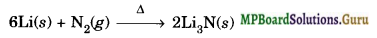(c) E° of any M2+/M electrode depends upon three factors : (i) enthalpy of vapourization, (ii) ionization enthalpy (iii) enthalpy of hydration. Since the combined effect of these factors is approximately the same for Ca, Sr and Ba, therefore, their electrode potential are nearly constant.

Question 27.
State as to why
(a) A solution of Na2CO3 is alkaline.
(b) Alkali metals are prepared by electrolysis of their fused chlorides.
(c) Sodium is found to be more useful than potassium.
Solution.
(a) An aqueous solution of sodium Carbonate has a large concentration of hydroxyl ions making it alkaline in nature
Na2CO3 + 2H2O → NaOH + H2CO3.

(b) Sodium is a very strong reducing agent, so it can not be extracted by the reduction of its ore (Chemical method). Thus, the best way to prepare sodium is by carrying out electrolysis of its molten salts containing impurities of calcium chloride.

(c) Sodium is relatively less reactive than potassium and hence its reactions with other substances can be better controlled and hence are safer to handle. Therefore, sodium is more useful than potassium.

Question 28.
Write balanced equations for the reactions between (a) Na2O2 and water
(b) KO2 and water
(c) Na2O and CO2.
Solution.
(a) Na2O2(s) + 2H2O(l) → 2NaOH(aq) + H2O2(aq)
(b) 2K02(S) + 2H2O(l) → 2KOH(aq) + H2O2(aq) + O2(g)
(c) Na2O + CO2 → Na2CO3.

Question 29.
How would you explain?
(i) BeO is insoluble but BeSO4 is soluble in water.
(ii) BaO is soluble but BaSO4 is insoluble in water.
(iii) Lil is more soluble than KI in ethanol.
Solution.
(i) BeO has higher lattice enthalpy than hydration enthalpy and hence is insoluble in water. BeSO4 on the contrary, is soluble because the lattice enthalpy is less due to bigger sulphate ion.

(ii) In barium oxide, BaO, due to larger size of barium ion the lattice enthalpy is less than hydration enthalpy and hence it is soluble in water. On the other hand, in BaSO4 due to larger size of barium as well as sulphate ion, the magnitude of hydration enthalpy is much smaller than the lattice enthalpy and hence it is insoluble in water.

(iii) In KI the chemical bond is ionic in character. On the other hand, due to small size of lithium ion and its high polarising power, the bond in Lil is predominantly covalent in character. Hence, Lil is more soluble than KI in ethanol.

Question 30.
Which of the alkali metals is having least melting point?
(a) Na
(b) K
(c) Rb
(d) Cs.
Solution.
As the atomic size of a metal increases, the strength of metallic bonding decreases and hence its melting point decreases. Since the size of Cs is the largest, therefore, its melting point is the lowest. Thus, choice (d) is correct.

Question 31.
Which of the following alkali metals gives hydrated salts?
(a) Li
(b) Na
(c) K
(d) Cs
Solution.
Among alkali metal ions, Li+ is the smallest in size. Therefore, it has the highest charge density and hence attracts the water molecules more strongly than any other alkali metal cation. Therefore, choice (a) is correct.

Question 32.
Thermally the most stable alkaline earth metal carbonate is:
(a) MgCO3
(b) CaCO3
(c) SrCO3
(d) BaCO3
Solution.
BaCO3 is thermally most stable because Ba2+ ion being larger in size is more stabilized by larger CO32- ion through formation of a stable lattice.### MP Board Class 11 Chemistry The s-Block Elements Important Questions and Answers

Question 1.
Why are the elements of group 1 called alkali metals?
Because they form strongly basic oxides and hydroxides.

Question 2.
Why are group 1 elements called s-block elements?
Because the last electron is added in s-orbital of outermost orbit.

Question 3.
What is charge density?
Charge density is the ratio of charge on ion to its size.

Question 4.
What is lattice energy?
Lattice energy is the energy required to break one mole of ionic solid into its constituent ions in gaseous state.

Question 5.
What is diagonal relationship?
The similarity between lightest element of one group and the second lightest element of the following group is called diagonal relationship.

Question 6.
Why alkali metals are extracted only by electrolytic method and not by chemical method?
They are highly electropositive and their cations cannot be reduced by chemical methods.

Question 7.
Sodium catches fire when dropped in water. Why?
Sodium reacts with water to produce H2 which catches fire due to heat generated in the reaction.

Question 8.
Out of Na and Li which is softer?
Sodium is softer than lithium.

Question 9.
Name the two most abundant alkali metals,
Sodium and potassium.Question 10.
What is the nature of lithium compounds?
Generally the lithium compounds are covalent.

Question 11.
Name the alkali metal that imparts yellow colour to Bunsen flame.
Potassium (K).

Question 12.
Which alkali metal carbonate evolves CO2 on being heated?
Li2CO3.

Question 13.
Why is the solution of alkali metals in liquid ammonia conducting in nature?
Due to the presence of ammoniated electrons and cations.

Question 14.
Name the metal which floats on water without any apparent reaction with it?
Lithium (Li).

Question 15.
Arrange the following in increasing order of their lattice energy:
LiF, NaF, KF, RbF and CsF.
CsF < RbF < KF < NaF < LiF.

Question 16.
An alkali metal hydride is used to fill the meteorological balloons. Name it.
Lithium hydride (LiH).

Question 17.
What is water glass?
Water glass is sodium silicate, Na2SiO3.

Question 18.
Name the alkali metal chloride that dissolves in alcohol.
Lithium Chloride (LiCl).Question 19.
Why is sodium kept under kerosene?
Sodium is readily oxidised if exposed to air or moisture and so is kept under kerosene.

Question 20.
Why are alkali metals soft and low melting?
Because of weak metallic bonding in the solid state which in turn is due to large atomic radii and having a single valence electron as compared to large number of available vacant orbitals.

Question 21.
Alkali metals are good reducing agents. Why?
(i) Alkali metals have very low ionization enthalpies. Hence, they can lose their valence electron readily and can bring about reduction, and behave as strong reducing agents.

Question 22.
Alkali metals do not form divalent ions. Why?
The second ionization enthalpy of alkali metals is very high because the M+ ions have stable noble gas configuration. Hence, alkali metals do not form divalent (M2+) ions.

Question 23.
Alkali metals are used in photoelectric cells. Why?
Alkali metals, because of their low ionization enthalpy, can lose electron easily on irradiation. Hence, they are used in photoelectric cells.

Question 24.
Potassium is more reactive than sodium. Why?
Reactivity of metals depends on their ionization enthalpy; smaller the ionization enthalpy, greater is the reactivity. Potassium has lower ionization enthalpy than so¬dium and hence is more reactive.

Question 25.
Sodium wire is used to dry benzene but cannot be used to dry ethanol.
Sodium metal removes moisture from benzene by reacting with water. However, ethanol cannot be dried by using sodium because it reacts with sodium.
2Na + C2H5OH → 2C2H5ONa + H2.

Question 26.
Pallets of potassium hydroxide become wet when exposed to air. Why?
Potassium hydroxide is highly deliquescent. It absorbs moisture from air and changes into solution. Thus, when potassium hydroxide pallets are exposed to air their surface becomes wet.Question 27.
During electrolysis of molten sodium chloride, calcium chloride or potassium chloride is added. Why?
Pure sodium chloride melts at 815°C which is close to the boiling point of sodium. Moreover, chlorine gas has corrosive action on the cell at high temperature. To lower the fusion temperature of sodium chloride to about 600°C, some calcium chloride or potassium chloride is added to it.

Question  28.
LiCl is predominantly covalent while NaCl is ionic.
Lithium-ion, due to its smaller size, has greater polarizing power than sodium ion. Hence, LiCl predominantly is covalent while NaCl is ionic.

Question 29.
Be(OH)2 is almost insoluble in water while Ba(OH)2 is soluble.
Due to small size and high charge density beryllium ion has high polarizing power. ‘ Hence, Ba(OH)2 is covalent in character and is almost insoluble in water. On the other hand, Ba2+ ion is larger in size and has small polarizing power. Therefore, Ba(OH)2 is ionic in character and is soluble in water.

Question 30.
Lithium on being heated in air mainly formes the monoxide and not peroxide. Why?
Li+ ions is smaller in size. It is stabilized more by smaller anion, oxide ion (O2-) as compared to larger anion, peroxide ion (O22-).

Question 31.
An aqueous solution of sodium carbonate is alkaline in nature.
In an aqueous solution sodium carbonate undergoes hydrolysis forming sodium hydroxide.
Na2CO3 + H2O → NaHCO3 + NaOH.

Question 32.
Describe the nature and properties of solutions of alkali metals in liquid ammonia.
All alkali metals dissolve in liquid ammonia giving deep blue solution. The solution contains ammoniated cations and ammoniated electrons as shown below :
M + (x + y) NH3→ M+(NH3)x + e(NH3)y
The blue colour of the solution is considered to be due to ammoniated electrons.
These solutions of alkali metals in liquid ammonia are very good conductors of electricity. Their conductivity is better than solution of any salt in any liquid. The conduction is mainly due to the presence of ammoniated electrons.Question 33.
LiCl has lower melting point than NaCl. Why?